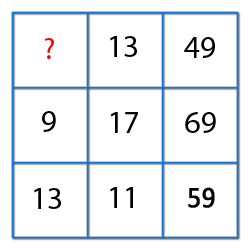﻿

### Reasoning

ISBT Reasoning for all banking PO,Clerk,IBPS PO,Railway,SSC,IAS,OAS Exams

##### Find the missing number.1) 4 2) 5 3) 8 4) 12 5) None of these
Explanation : Second Row, 2 x 9 + 3 x 17 = 69.

Third Row, 2 x 13 + 3 x 11 = 59.

Let , missing number be x,
So, 2x + 3 x 13 = 49.

or, x = 5.View Answer
##### Direction :Read the following information and give the answer. A, B, C, D, E, F and G are sitting in a row facing North : 1. F is to the immediate right of E.    2. E is 4th to the right of G.    3. C is the neighbour of B and D    4. Person who is third to the left of D is at one of ends.Who are to the left of C ?
 1) Only B 2) G, B and D 3) G and B 4) D, E, F and A 5) None of these
Explanation :View Answer
##### Direction : Each of these questions are based on the information given below : I. Eight persons E, F, G, H, I, J, K and L are seated around a square table - two on each side. II. There are 3 ladies who are not seated next to each other. III. J is between L and F. IV. G is between I and F. V. H, a lady member is second to the left of J. VI. F, a male member is seated opposite to E, a lady member. VII. There is a lady member between F and I.Who is sitting third to the left of J ?
 1) K 2) l 3) I 4) M 5) None of these
Explanation :So, K is third to the left of J.View Answer
##### Direction : Each of these questions are based on the information given below : I. Eight persons E, F, G, H, I, J, K and L are seated around a square table - two on each side. II. There are 3 ladies who are not seated next to each other. III. J is between L and F. IV. G is between I and F. V. H, a lady member is second to the left of J. VI. F, a male member is seated opposite to E, a lady member. VII. There is a lady member between F and I.Who among the following is to the immediate left of F ?
 1) G 2) I 3) J 4) H 5) None of these
Explanation :J is to the immediate left of F.
J is to the immediate left of F.View Answer
##### Direction : Each of these questions is based on the following information:  A + B means A is the mother of B   A - B means A is the sister of B  A * B means A is the father of B  A ÷ B means A is the brother of BWhich of the following means that N is the maternal uncle of M ?
 1) N ÷ P - L + E - M 2) N - Y + A ÷ M 3) M - Y * P - N 4) N ÷ C + F * M 5) None of these
Answer : N ÷ P - L + E - M
Explanation :
N  ÷ P  means  N is the brother of P
P - L  means P is the sister of L
L + E  means L is the mother of E
E - M means E is the sister of M.
Hence, L is the mother of M, P is the maternal aunt of M and N is the maternal uncle of M.View Answer
##### "A"walks 20 km towards North. He turns left and walks 40 km. He again turns left and walks 20 km. Finally he moves 20 km after turning to the left. He is facing towards in which direction to his starting point ?
 1) East 2) West 3) North 4) South 5) None of these
Explanation :So, he is facing towards east direction.View Answer
##### Directions : Read the following character sequence carefully and then answer the questions given below it: D E 5 B F K 8 P M T 7 H O 6 S R Q 4 X C 3 A Z W 2 LWhich of the following will be the third to the right of 16th character from your right ?
 1) H 2) O 3) 6 4) S 5) R
Explanation : 3rd to the right of 16th from right = (16-3=) 13th from right = 6View Answer
##### Directions : Read the following character sequence carefully and then answer the questions given below it: D E 5 B F K 8 P M T 7 H O 6 S R Q 4 X C 3 A Z W 2 LIf every alternate character in the above sequence, starting from K on wards, is dropped, which of the following characters will be third to the right of ninth character from the left ?
 1) S 2) R 3) Q 4) 4 5) X
Explanation :
3rd to the right of 9th from the left = (9+3=) 12th from the left .
When every alternate character, starting from K, is dropped
J D E 5 B F 8 M 7 O S O X . . . . .View Answer
##### Directions : Read the following character sequence carefully and then answer the questions given below it: D E 5 B F K 8 P M T 7 H O 6 S R Q 4 X C 3 A Z W 2 LWhat will be the ninth character to the left of nineteenth character from the left in the above alphabet sequence ?
 1) M 2) T 3) 7 4) H 5) OView AnswerView Answer